# Angles

Determine the interior angles of a rhombus with area 319.1 cm2 and perimeter 72 cm.

Result

α =  80 °
β =  100 °
γ =  80 °
δ =  100 °

#### Solution:Leave us a comment of example and its solution (i.e. if it is still somewhat unclear...):

Showing 0 comments:Be the first to comment!#### To solve this example are needed these knowledge from mathematics:

Do you want to convert length units? Most natural application of trigonometry and trigonometric functions is a calculation of the triangles. Common and less common calculations of different types of triangles offers our triangle calculator. Word trigonometry comes from Greek and literally means triangle calculation.

## Next similar examples:

1. Triangle SAAThe triangle has one side long 71 m and its two internal angles is 60°. Calculate the perimeter and area of the triangle.
2. Right triangleLadder 16 feet reaches up 14 feet on a house wall. The 90-degree angle at the base of the house and wall. What are the other two angles or the length of the leg of the yard?
3. Rhombus A2p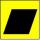Rhombus area is 13 cm2 and its height is 5cm long . Determine a perimeter of this rhombus.
4. Jewel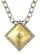Rhombus-shaped jewel have area of 93 mm2 and the edge in long 13.2 mm. Calculate the size of rhombus acute angle.
5. Rhomboid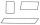Calculate the circumference and area of the rhomboid with sides 20 and 10, with their angle 20°.
6. Rail turn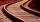The curve radius on the railway line is 532 m . Length of rail track on it is 818 m. How many degrees is the angle ASB, if A and B are boundary points and S is center of arc curve.
7. Rhombus 4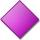Circumference of the rhombus is 44 cm, its height is 89 mm long. Calculate its content area.
8. RhombusPQRS is a rhombus. Given that PQ=3 cm & height of rhombus is given 2 cm. Calculate its area.
9. Rhombus diagonalArea of rhombus is 224. One diagonal measures 33, find length of other diagonal.
10. SlopeFind the slope of the line: x=t and y=1+t.
11. An angleAn angle x is opposite side AB which is 10, and side AC is 15 which is hypotenuse side in triangle ABC. Calculate angle x.
12. Diagonals of the rhombus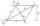Calculate height of rhombus whose diagonals are 12 cm and 19 cm.
13. Roof tilesThe roof has a trapezoidal shape with bases of 15 m and 10 m, height of roof is 4 meters. How many tiles will need if on 1 m2 should be used 8 tiles?
14. Circular lawnAround a circular lawn area is 2 m wide sidewalk. The outer edge of the sidewalk is curb whose width is 2 m. Curbstone and the inner side of the sidewalk together form a concentric circles. Calculate the area of the circular lawn and the result round to 1
15. AceThe length of segment AB is 24 cm and the point M and N divided it into thirds. Calculate the circumference and area of this shape.
16. Land areas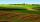Two land areas is 244 m2. The first parcel is 40 m2 less than twice of the second one. What have acreage of each parcel?
17. Estate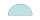Semicircle estate must be fence. The straight section has 26 meters long fence. How many meters of fence should buy?